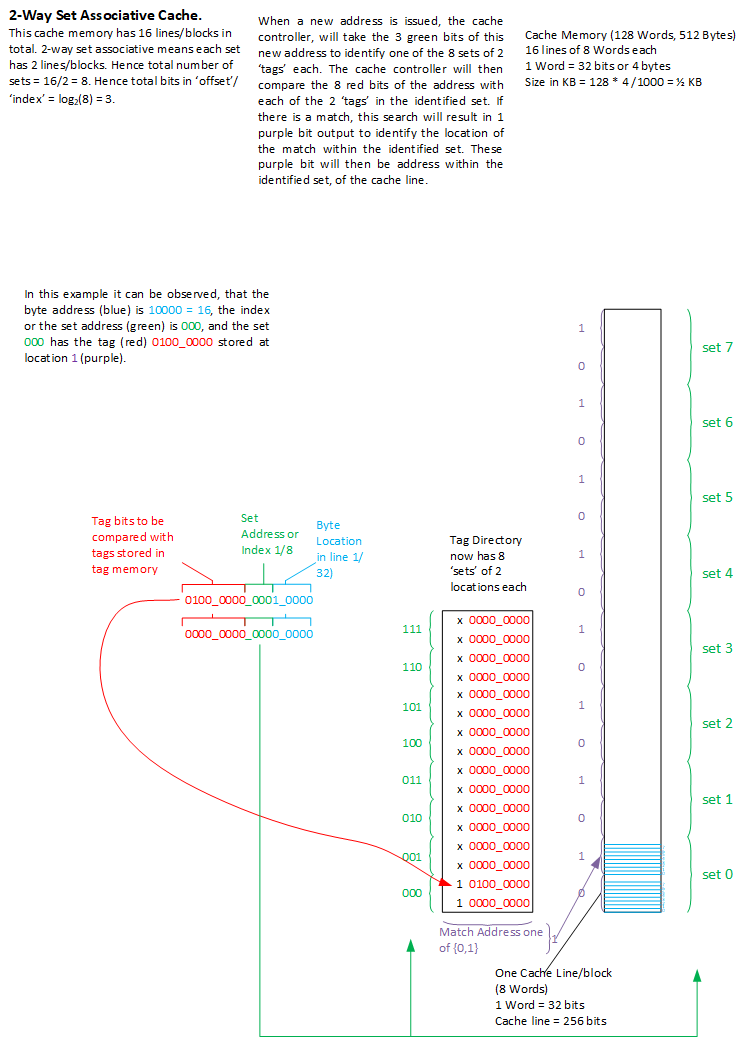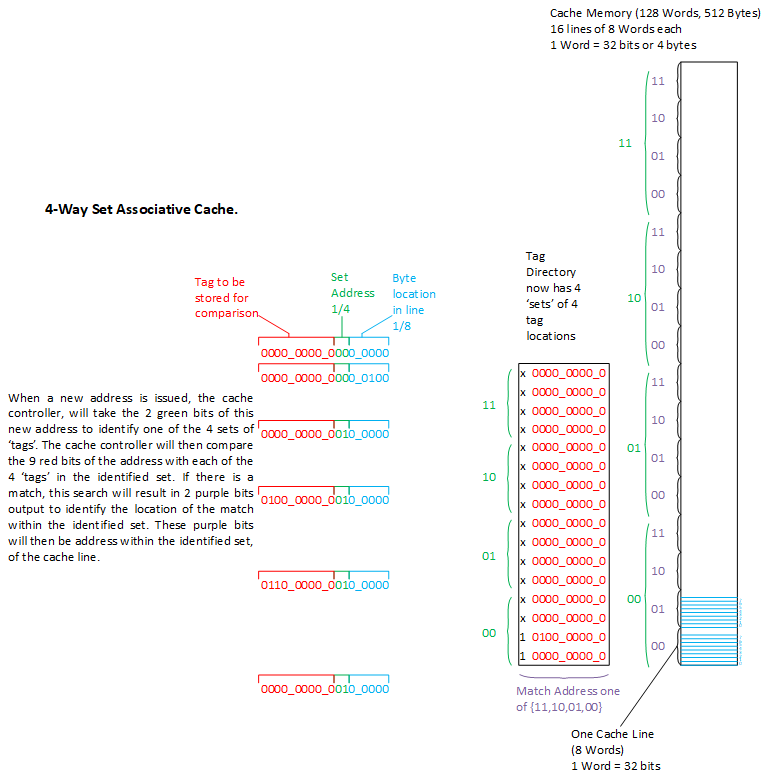Cache Memory Tutorial - 4
-Aviral Mittal (avimit att yhaoo dat cam)

Cache Memories - 4: Associativity in Cache memories.
In the previous Section(s), it was explained how caches are organized and what is a direct-mapped cache. In this chapter we will learn about what is called 'associativity in caches'.
This chapter is about structure and organization of cache memories.

In Direct Mapped cache organization, the SET address identifies the TAG location in TAG RAM and also the location of cache line in DATA RAM.
What if the 'SET' address identifies a 'set' of cache lines in memory, and a 'set' of TAG locations in TAG memory.
This actually means that the cache-organization now changes, such that a 'set' is now a collection of multiple cache lines (in powers of 2)
e.g a set can identify a collection of collection of say 2 cache lines, or 4 cache lines or 8 cache lines and so on and so forth.
If the cache organization is such that the 'SET' address identifies a set of '2' cache lines, the cache is said to be 2-way set associative.
If the cache organization is such that the 'SET' address identifies a set of '4' cache lines, the cache is said to be 4-way set associative and so on and so forth.

Example: 2-way set associative cache:

Let us take an example of a very small cache:
Memory size = 0.5 KB
Cache line = 32 bytes (256 bits).

Offset address              = Log2(cache line size in bytes)                 = Log2(32) = 5 bits
Total number of cache lines = memory size / cache line size                  = 512/32   = 16
Number of Sets              = Number of cache lines / Associativity          = 16/2     = 8
Set address width           = Log2(Number of sets) = Log2(8)                 = 3
TAG width                   = Full address width - SET width - Offset width  = 16-3-5   = 8 Bits

Following diagram shows the organization of 2-way set associative 0.5KB cache memory with 32 bytes cache line size.How does this N-way set associativity works? let us answer this by the example above (2-way set associative cache).

Example: 4-way set associative cache:
Memory size = 0.5 KB
Cache line = 32 bytes (256 bits).
Associativity = 4-Way

Offset address              = Log2(cache line size in bytes)                 = Log2(32)  = 5 bits
Total number of cache lines = memory size / cache line size                  = 512/32    = 16
Number of Sets              = Number of cache lines / Associativity          = 16/4      = 4.
Set address width           = Log2(Number of sets) = Log2(4)                 = 2.
TAG width                   = Full address width - SET width - Offset width  = 16 - 2 -5 = 9 Bits.Example to calculate the TAG width, SET Address width, Offset bits:

Address size = 32 bits, cache line size is 256 bits (32 bytes), cache size is 1 MB. Associativity = 4-way associative.

Width of offset = Log2(32) = 5.
Number of cache lines = 1000 KB/32 = 32 K.
Number of sets = 32K/4(4 way associative) = 8K, hence the size of SET address in bits = Log2(8K) = 13.
Hence the width of TAG = 32 - 13 (set width) - 5 (offset width ) = 14 bits.
The Result:
TAG = 14 bits
SET = 13 bits
OFFSET = 5 bits.

Conclusion:
A N-Way set associative cache can store a cache line in any of the N-locations with in a 'SET' of cache lines identified by the SET address.

<= Cache Organization Fundamentals                            Next => No Next

Cache Organizations:
Direct-Mapped-Cache
4-Way-Set-Associative Cache
2-Way-Set-Associative Cache

Keywords:
What is a cache memory
How does a cache memory work
What is Cache Miss
What is Cache Hit
What is 'tag'.